GeometryShowing results 31 to 40 of 51, on page 4 of 6 Area Coordinate Systems Curves and Shapes Length/Perimeter Volume
 DESCRIPTION EQUATION Equation of an Ellipsoid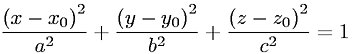Equation of an Elliptic Cone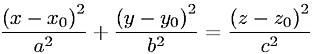Equation of an Elliptic CylinderEquation of an Elliptic Paraboloid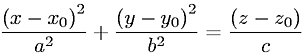Perimeter of a Circle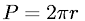Perimeter of a RectanglePerimeter of a Regular PolygonPerimeter of a Square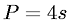Perimeter of a TriangleSpiral of Archimedes (Archimedean Spiral) in Polar Coordinates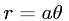Go to page: 1  2  3  4  5  6